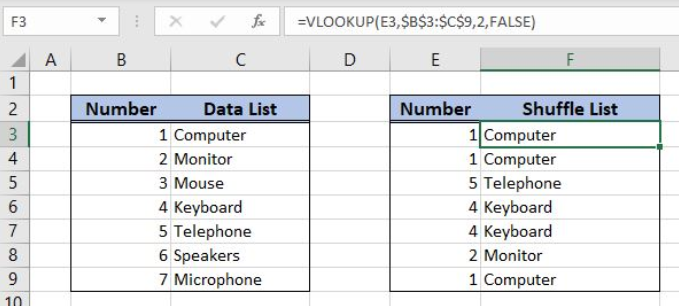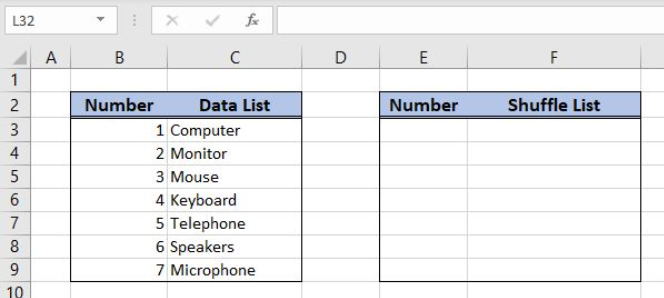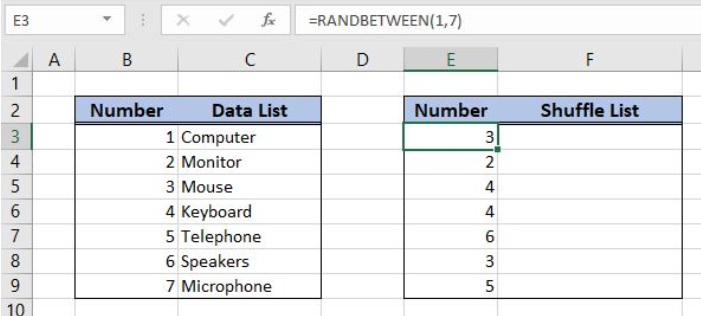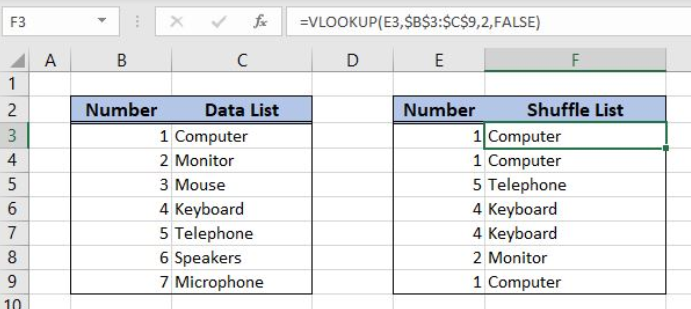Get instant live expert help with Excel or Google Sheets“My Excelchat expert helped me in less than 20 minutes, saving me what would have been 5 hours of work!”

#### Post your problem and you’ll get expert help in seconds.

Your message must be at least 40 characters
Our professional experts are available now. Your privacy is guaranteed.

# Here is How You Can Shuffle a List in Excel

Excel allows a user to shuffle a list using the RANDBETWEEN and VLOOKUP functions. This step by step tutorial will assist all levels of Excel users to find out how to shuffle a list in Excel.Figure 1. The final result of the formula

## Syntax of the RANDBETWEEN Formula

The generic formula for the RANDBETWEEN function is:

`=RANDBETWEEN(bottom, top)`

The parameters of the RANDBETWEEN function are:

• bottom – a value from which we want to get a random value
• top – a value to which we want to get a random value.

## Syntax of the VLOOKUP formula

The generic formula for the VLOOKUP function is:

`=VLOOKUP(lookup_value, table_array, col_index_num, range_lookup)`

The parameters of the VLOOKUP function are:

• lookup_value – a value that we want to find in a table_array
• table_array – a range in which we want to lookup
• col_index_num – a column number in table_array from which we would like to get a value
• range_lookup – default value is FALSE. This means that we want to find an exact match for a lookup value.

## Setting up Our Data for the Example

Let’s look at the structure of data that we will use. In the first table, we have a list of data with numbers. In column B we have numbers and in column C data items. In the second table, we want to get the same list but with randomized data items.Figure 2. Data that we will use in the example

## Shuffle a Data List Using the Formula

To shuffle the data list, we first need to randomize the numbers using the RANDBETWEEN function. After that, we can lookup for data using the VLOOKUP function.

The formula for RANDBETWEEN in E3 looks like:

`=RANDBETWEEN(1, 7)`

The parameter bottom of the RANDBETWEEN function is 1 and the top is 7, as we have 7 items in the table.

To apply the formula, we need to follow these steps:

• Select cell E3 and click on it
• Insert the formula: `=RANDBETWEEN(1, 7)`
• Press enter
• Drag the formula down to the other cells in the column by clicking and dragging the little “+” icon at the bottom-right of the cell.Figure 3. Using the RANDBETWEEN formula

After creating this formula, we can see that in column E we have numbers 1-7 randomized. Now we can lookup for the data in column C based on numbers matching.

The formula for VLOOKUP in F3 looks like:

`=VLOOKUP(E3, \$B\$3:\$C\$9, 2, FALSE)`

The lookup_value parameter is the cell E3. The table_array is \$B\$3:\$C\$9. We need to fix the range as it’s not changing when the formula is copied. The col_index_num is 2, as we want to get the value from the second column of the range. The range_lookup is FALSE.Figure 4. Using the VLOOKUP formula

In the cell E2 we got randomized number 1. The according value for 5 in the first table is “Computer”, which is the result in F3. As we can see the whole list from the first table is shuffled in the F3:F9 range.

Most of the time, the problem you will need to solve will be more complex than a simple application of a formula or function. If you want to save hours of research and frustration, try our live Excelchat service! Our Excel Experts are available 24/7 to answer any Excel question you may have. We guarantee a connection within 30 seconds and a customized solution within 20 minutes.

Solution examplesI filtered data in column A (it is labeled ID #) of a data set. On my next worksheet, column A is also the ID #, but it is a different data set. I want to filter the ID #'s the same for the two sheets, but how do I do that being that I am working with two different sets of data?
Solved by V. H. in 22 minsI'm looking for a formula or format for if there is data in c4 on a sheet... It adds extra information underneath
Solved by X. E. in 20 minshi, my vlookup wont work in some rows but does in others. its temperemental. e.g it will work on rows 10 to 15, then not row 16 and 17, but the will for the rest.
Solved by K. Q. in 21 minsI have a pivot table in which per order (on the rows) stands how much products they ordered per size (on the columns). I want to determine which combinations of quantities of sizes people order. And I want to count these combinations.
Solved by E. W. in 60 minsI have 500 numbers in column A with aproximatly 15digits. In column B I have 5 numbers with 6 digits Can a find a formula wich can transfer all numbers from A column wich start with some 6 digitsa from B column
Solved by V. L. in 32 mins